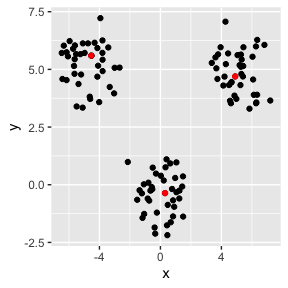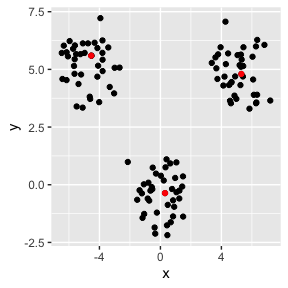# Almost Linear-Time k-Medoids Clustering

## Introduction

banditpam is an R package that lets you do $$k$$-mediods clustering efficiently as described in Tiwari, et. al. (2020).

We illustrate with a simple example using simulated data from a Gaussian Mixture Model with the the following means: $$(0, 0)$$, $$(-5, 5)$$ and $$(5, 5)$$.

set.seed(10)
n_per_cluster <- 40
means <- list(c(0, 0), c(-5, 5), c(5, 5))
X <- do.call(rbind, lapply(means, MASS::mvrnorm, n = n_per_cluster, Sigma = diag(2)))

Let’s cluster the observations in this X matrix using 3 clusters. The first step is to create a KMedoids object:

obj <- KMedoids$new(k = 3) Next we fit the data with a specified loss, $$l_2$$ here. A good habit is to set the seed before fitting for reproducibility. set.seed(198) obj$fit(data = X, loss = "l2")

And we can now extract the medoid observation indices.

med_indices <- obj$get_medoids_final() A plot shows the results where we color the medoids in red. d <- as.data.frame(X); names(d) <- c("x", "y") dd <- d[med_indices, ] ggplot(data = d) + geom_point(aes(x, y)) + geom_point(aes(x, y), data = dd, color = "red")Clustering with 3-mediods with L2 loss We can also change the loss function and see how the mediods change. obj$fit(data = X, loss = "l1")  # L1 loss
med_indices <- obj$get_medoids_final()Clustering with 3-mediods with L1 loss One can query some performance statistics too; see help on KMedoids. obj$get_statistic("dist_computations") # no of dist computations
#>  32517
obj\$get_statistic("cache_misses") #  no of cache misses
#>  0